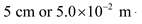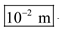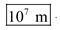# Give an order-of-magnitude estimate for the length in meters of the following:

Give an order-of-magnitude estimate for the length in meters of the following: (a) a person; (b) a fly;
( c) a car; (d) a 747 airplane; (e) an interstate freeway stretching coast-to-coast.

(a)
The average height of a typical person is around 1.80 m.
Thus, the order of magnitude in meters to the nearest power of 10 is 1m

(b)
The length of the fly is aroundThus, the order of magnitude in meters to the nearest power of 10 is( c)
The length of the car is in between 6m and 11m
Thus, the order of magnitude in meters to the nearest power of 10 is 10m.

(d)
The length of 747 airplane is about 100 m
Thus, the order of magnitude in meters to the nearest power of 10 is 100m.

(e)
The length of the interstellar freeway is about.
Thus, the order of magnitude in meters to the nearest power of 10 is